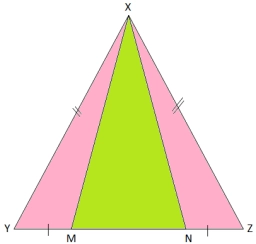# Angles - triangle

Find the measure of the angles of the triangle ABC, if: the measure of the angle A=the measure of the angle C, and the measure of the angle B =½ of the measure of the angle C.

A =  72 °
B =  36 °
C =  72 °

### Step-by-step explanation:Did you find an error or inaccuracy? Feel free to write us. Thank you!

Tips for related online calculators
Do you have a linear equation or system of equations and looking for its solution? Or do you have a quadratic equation?